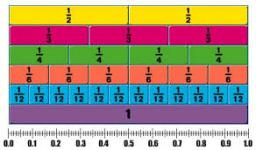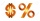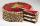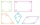# Ten fractions

Write ten fractions between 1/3 and 2/3

a1 =  0.3667
a2 =  0.3833
a3 =  0.4
a4 =  0.4167
a5 =  0.4333
a6 =  0.45
a7 =  0.4667
a8 =  0.4833
a9 =  0.5
a10 =  0.5167

### Step-by-step explanation:

${a}_{1}=110\mathrm{/}300=\frac{11}{30}=0.3667$
${a}_{2}=115\mathrm{/}300=\frac{23}{60}=0.3833$
${a}_{3}=120\mathrm{/}300=\frac{2}{5}=0.4$
${a}_{4}=125\mathrm{/}300=\frac{5}{12}=0.4167$
${a}_{5}=130\mathrm{/}300=\frac{13}{30}=0.4333$
${a}_{6}=135\mathrm{/}300=\frac{9}{20}=0.45$
${a}_{7}=140\mathrm{/}300=\frac{7}{15}=0.4667$
${a}_{8}=145\mathrm{/}300=\frac{29}{60}=0.4833$
${a}_{9}=150\mathrm{/}300=\frac{1}{2}=0.5$
${a}_{10}=155\mathrm{/}300=\frac{31}{60}=0.5167$Did you find an error or inaccuracy? Feel free to write us. Thank you!Tips to related online calculators
Need help to calculate sum, simplify or multiply fractions? Try our fraction calculator.

## Related math problems and questions:

• FractionsSort fractions z1 = (6)/(11); z2 = (10)/(21); z3 = (19)/(22) by its size. Result write as three serial numbers 1,2,3.
• The temperatureThe temperature at 1:00 was 10 F. Between 1:00 and 2:00, the temperature dropped 15F. Between 2:00 and 3:00, the temperature rose 3F. What is the temperature at 3:00?Why does 1 3/4 + 2 9/10 equal 4.65? How do you solve this?
• 76% is76% is between which of the following two numbers. 1) 1/5 & 1/4. 2) 1/3 & 1/2 3) 2/3 & 7/10 4) 3/4 & 5/6
• Evaluate mixed expressionsWhich of the following is equal to 4 and 2 over 3 divided by 3 and 1 over 2? A. 4 and 2 over 3 times 3 and 2 over 1 B. 14 over 3 times 2 over 7 C. 14 over 3 times 7 over 2 D. 42 over 3 times 2 over 31
• Fractions and mixed numerals(a) Convert the following mixed numbers to improper fractions. i. 3 5/8 ii. 7 7/6 (b) Convert the following improper fraction to a mixed number. i. 13/4 ii. 78/5 (c) Simplify these fractions to their lowest terms. i. 36/42 ii. 27/45 2. evaluate the follow
• Expression plus minusEvaluate expression: (-1)2 . 12 – 6 : 3 + (-3) . (-2) + 22 – (-3) . 2Add two mixed fractions: 2 4/6 + 1 3/6
• What is 9What is the value of x in the proportion 2 and one-fourth over x = 1 and one-half over 3 and three-fifths? 2 and two-fifths 5 and two-fifths 8 and 1 over 10 12 and 3 over 20
• Cake fractionsThomas ate 1/3 of cake, Bohouš of the rest of the cake ate 2/5. What fraction of cake left over for others?
• Sum three fractionsWork out the sum of 1/4, 1/5 and 3/10.
• Evaluate fractionsThe difference  of 5 1/2 and 2/3 is added to the product of 5/6 and 1/2
• ProductResult of the product of the numbers 1, 2, 3, 1, 2, 0 is:
• Mixed numbersRewrite mixed numbers, so the fractions have the same denominator: 5 1/5 - 2 2/3
• The perimeter 2The perimeter of the quadrilateral a = 1m b = 14/5m c = 2 3/10m d = 1 4/5m?
• PoundsThree pounds subtract 1/3 of a pound.
• SchoolThere are 150 pupils in grade 5 . 2/3 of them are female. By what fractions are the males?